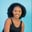Related Tags

golang
communitycreator

# How to use the strconv.AppendFloat() function in GolangMaria Elijah

### Golang strconv.AppendFloat()

The strconv package’s AppendFloat() method in Golang is used to append the string form of the specified floating-point number f (as generated by the FormatFloat() function) to dst and return the extended buffer. The parameter dst is of type []byte and f is of type float64.

### Syntax

func AppendFloat(
dst []byte,
f float64,
fmt byte,
prec, bitSize int) []byte


### Parameters

• dst: This is a byte array to which the floating-point number is to be appended as a string.
• f: This is the floating-point number to be appended to dst.
• fmt: This is used to specify formatting.
• prec: This is the precision of the floating-point number which will be appended to the string.
• bitSize: This is the bit size (32 for float32, 64 for float64).

### Return value

The function AppendFloat() returns the extended buffer after appending the given floating-point value.

### Code

The following code shows how to implement the strconv.AppendFloat() in Golang.


// Using strconv.AppendFloat() Function

package main

import (
"fmt"
"strconv"
)

func main() {
// Declaring and assigning value
x := []byte("Some text with a float64: ")
fmt.Println("Before AppendFloat():", string(x))

// Appending float-point value
// prec, fmt, and bitsize
x = strconv.AppendFloat(x, 56.37781, 'E', -1, 64)
fmt.Println("After AppendFloat():",string(x))
fmt.Println()

// Declaring and assigning value
y := []byte("Some text with a float64: ")
fmt.Println("Before AppendFloat():", string(y))

// Appending float-point value
// prec, fmt, and bitsize
y = strconv.AppendFloat(x, 56.37781, 'g', 2, 32)
fmt.Println("After AppendFloat():",string(y))
fmt.Println()
}
Using the AppendFloat() function

### Code explanation

• Line 4: We add the main package.
• Lines 6–9: We import the necessary packages.
• Lines 11–31: We define the main() function, variables x and y of Float64 and Float32 type, respectively, and assign a value to each. Next, We pass the variables to the AppendFloat() function which appends the string form of the specified floating-point number f according to the fmt, prec, and bitSize parameters.

RELATED TAGS

golang
communitycreator

CONTRIBUTORMaria Elijah
RELATED COURSES

View all Courses

Keep Exploring

Learn in-demand tech skills in half the time